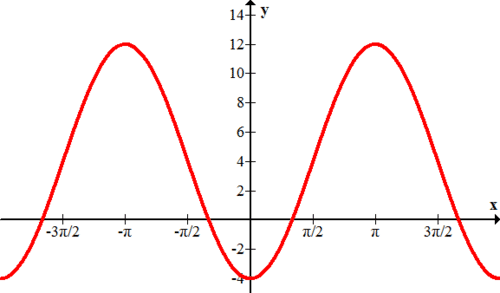5.4: Vertical Shift of Sinusoidal Functions

•• Contributed by CK12
• CK12

Your knowledge of transformations, specifically vertical shift, apply directly to sinusoidal functions.  In practice, sketching shifted sine and cosine functions requires greater attention to detail and more careful labeling than other functions.  Can you describe the following transformation in words?

$$f(x)=\sin x \rightarrow g(x)=-3 \sin x-4$$

In what order do the reflection, stretch and shift occur?  Is there a difference?

Vertical Shift of Sinusoidal Functions

The general form of a sinusoidal function is:

$$f(x)=\pm a \cdot \sin (b(x+c))+d$$

Recall that $$a$$ controls amplitude and the $$\pm$$ controls reflection. Here you will see how $$d$$ controls the vertical shift.

The most straightforward way to think about vertical shift of sinusoidal functions is to focus on the sinusoidal axis, the horizontal line running through the middle of the sine or cosine wave. At the start of the problem identify the vertical shift and immediately draw the new sinusoidal axis. Then proceed to graph amplitude and reflection about that axis as opposed to the $$x$$ axis.

The graphs of the following three functions are shown below:

$$f(x)=\sin x+3$$
$$g(x)=\sin x-2$$
$$h(x)=\sin x+\frac{1}{2}$$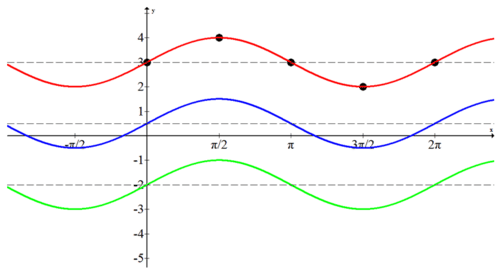To draw these graphs, the new sinusoidal axis for each graph is drawn first. Then, a complete sine wave for each one is drawn. Note the five important points that separate each quadrant to help to get a clear sense of the graph. There are no reflections in these graphs and they all have an amplitude of 1. Right now every cycle starts at 0 and ends at $$2 \pi$$ but this will not always be the case.

Watch the portions of the following video focused on vertical translations:

Examples

Example 1

Earlier, you were asked which order vertical shift and reflection should be performed in and if it matters. The following transformation can be described as follows.

$$f(x)=\sin x \rightarrow g(x)=-3 \sin x-4$$

Describe the stretching and reflecting first and then the vertical shift.  This is the most logical way to discuss the transformation verbally because then the numbers like 3 and -4 can be explicitly identified in the graph.

The order in describing the transformation matters.  When describing vertical transformations it is most intuitive to simply describe the transformations in the same order as the order of operations.

Example 2

Identify the equation of the following transformed cosine graph.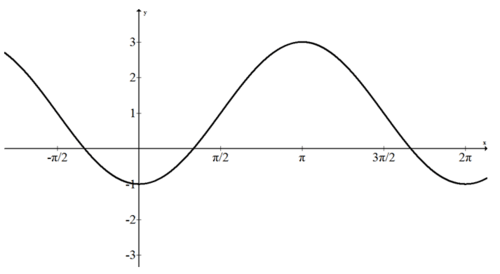since there is no sinusoidal axis given, you must determine the vertical shift, stretch and reflection. The peak occurs at $$(\pi, 3)$$ and the trough occurs at (0,-1) so the horizontal line directly between +3 and -1 is $$y=1$$. since the sinusoidal axis has been shifted up by one unit $$d=1$$. From this height, the graph goes two above and two below which means that the amplitude is 2 . since this cosine graph starts its cycle at (0,-1) which is a lowest point, it is a negative cosine. The function is $$f(x)=-2 \cos x+1$$

Example 3

Transform the following sine graph in two ways. First, transform the sine graph by shifting it vertically up 1 unit and then stretching it vertically by a factor of 2 units. Second, transform the sine graph by stretching it vertically by a factor of 2 units and then shifting it vertically up 1 unit.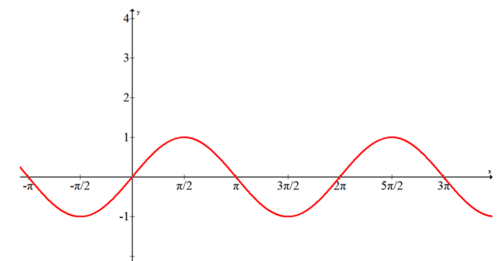When doing ordered transformations it is good to show where you start and where you end up so that you can effectively compare and contrast the outcomes.  See how both transformations start with a regular sine wave.  The two columns represent the sequence of transformations that produce different outcomes.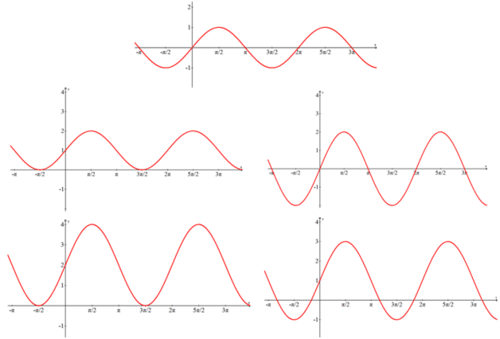Example 4

What equation models the following graph?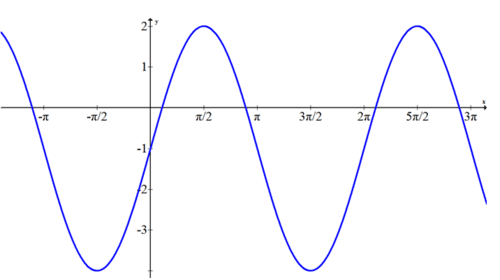$$f(x)=3 \cdot \sin x-1$$

Example 5

Graph the following function: $$f(x)=-2 \cdot \cos x+1$$

First draw the horizontal sinusoidal axis and identify the five main points for the cosine wave.  Be careful to note that the amplitude is 2 and the cosine wave starts and ends at a low point because of the negative sign.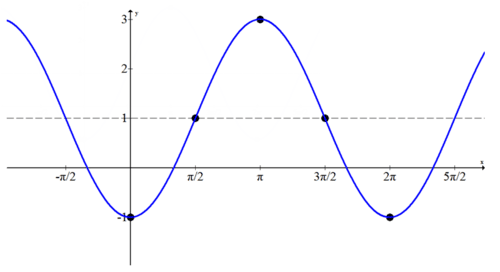Review

Graph each of the following functions that have undergone a vertical stretch, reflection, and/or a vertical shift.

1. $$f(x)=-2 \sin x+4$$

2. $$g(x)=\frac{1}{2} \cos x-1$$

3. $$h(x)=3 \sin x+2$$

4. $$j(x)=-1.5 \cos x+\frac{1}{2}$$

5. $$k(x)=\frac{2}{3} \sin x-3$$

Find the minimum and maximum values of each of the following functions.

6. $$f(x)=-3 \sin x+1$$

7. $$g(x)=2 \cos x-4$$

8. $$h(x)=\frac{1}{2} \sin x+1$$

9. $$j(x)=-\cos x+5$$

10. $$k(x)=\sin (x)-1$$

Give the equation of each function graphed below.

11.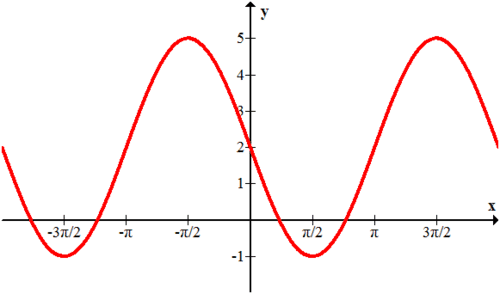12.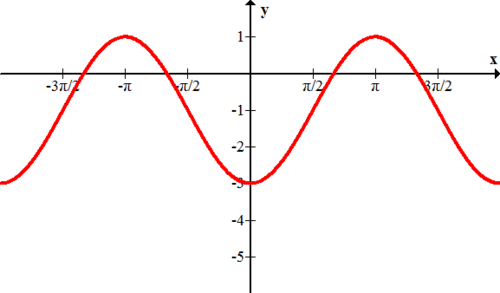13.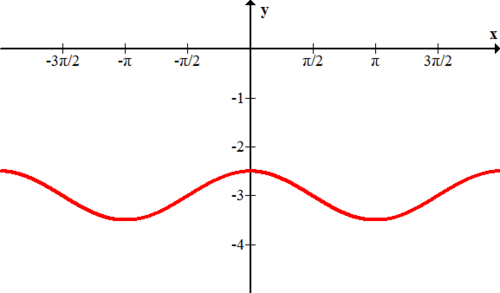14.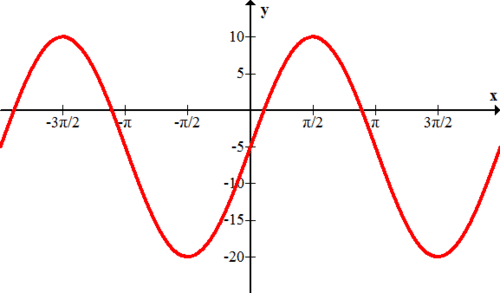15.